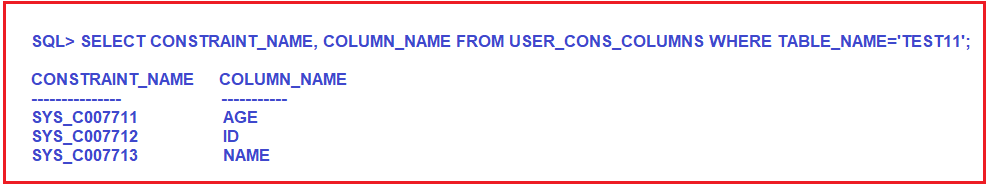# How to Rename a Constraint in Oracle

## How to Rename a Constraint in Oracle with Examples

In this article, I am going to discuss How to Rename a Constraint in Oracle with Examples. Please read our previous article where we discussed How to add and drop constraints from an existing table in Oracle with Examples.

##### How to Rename a Constraint in Oracle?

We need to use the following syntax to rename a constraint in oracle.

Syntax: ALTER TABLE <TN> RENAME CONSTRAINT < OLD CONSTRAINT NAME> TO <NEW CONSTRAINT NAME>;

##### Example:

Here we are creating the constraint with the predefined name which is provided by the Oracle server.

```CREATE TABLE TEST11
(
Id INT PRIMARY KEY,
Name VARCHAR2(20) UNIQUE,
Age INT CHECK (Age >= 18)
);
```

Once you execute the above CREATE TABLE statement, the TEST11 table will be created with three constraints. You can use the following SQL query, to get the name of the constraints which are created by the Oracle server on the TEST11 table.

`SELECT CONSTRAINT_NAME, COLUMN_NAME FROM USER_CONS_COLUMNS WHERE TABLE_NAME='TEST11';`

When you execute the above query, you will get the following output.Here, SYS_C007711 is the Check constraint, SYS_C007712 is the Primary key Constraint, and SYS_C007713 is the unique key constraint. Let us update these constraint names by giving user-defined meaningful names by executing the following queries.

```ALTER TABLE TEST11 RENAME CONSTRAINT SYS_C007711 TO AGE_CHECK;
ALTER TABLE TEST11 RENAME CONSTRAINT SYS_C007712 TO ID_PK;
ALTER TABLE TEST11 RENAME CONSTRAINT SYS_C007713 TO NAME_UK;```

Once you execute the above query, now you can verify the constraint names by executing the following SELECT statement.

SELECT CONSTRAINT_NAME FROM USER_CONS_COLUMNS WHERE TABLE_NAME=’TEST11′;

Once you execute the above query, you will get the following output.In the next article, I am going to discuss How to Enable and Disable Constraints in Oracle with Examples. Here, in this article, I try to explain How to Rename a constraint in Oracle with Examples and I hope you enjoy this article